Function Repository Resource:

# UnitNormal

Compute the unit normal of a surface

Contributed by: Alfred Gray
 ResourceFunction["UnitNormal"][s,{u,v}] gives the unit normal vector field to the surface s parametrized by variables u,v.

## Details and Options

ResourceFunction["UnitNormal"] effectively computes the Gauss map of s that maps every point on a surface to a corresponding point on the unit sphere.

## Examples

### Basic Examples (3)

Define a torus surface:

 In:=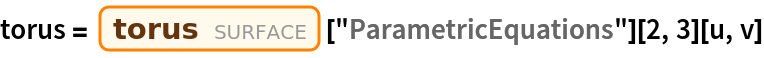Out=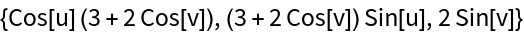Get the unit normals of the surface:

 In:=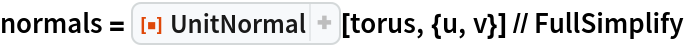Out=Plot the vector field:

 In:=Out=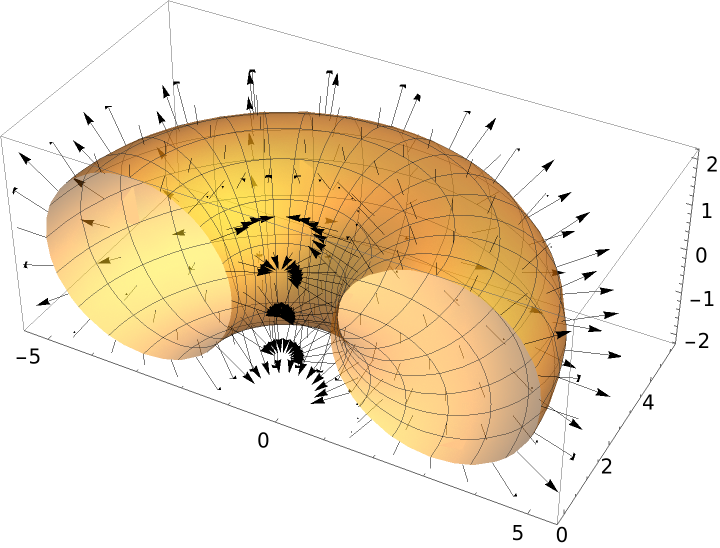### Scope (2)

Define a Möbius strip:

 In:=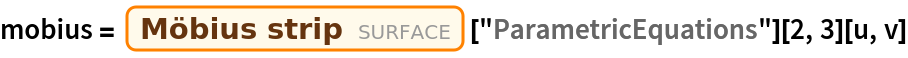Out=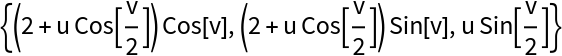Get the unit normals of the surface:

 In:=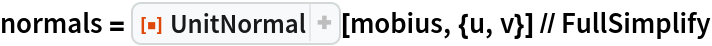Out=A Gauss map is not well defined on the whole surface, as unit normal vectors are only defined up to sign and there is no way to consistently decide the direction of the normal vector:

 In:=Out=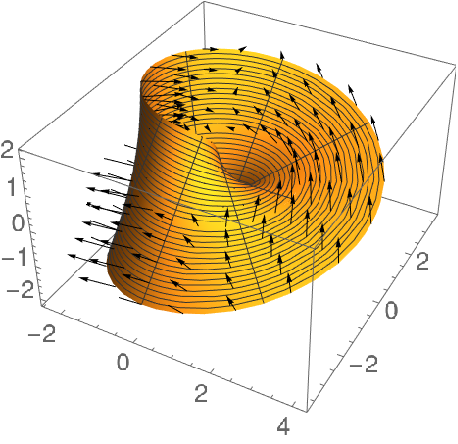Define a sine surface:

 In:=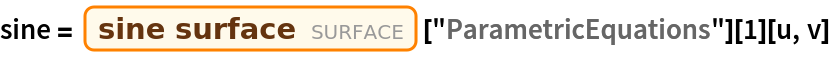Out=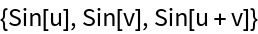Plot the sine surface:

 In:=Out=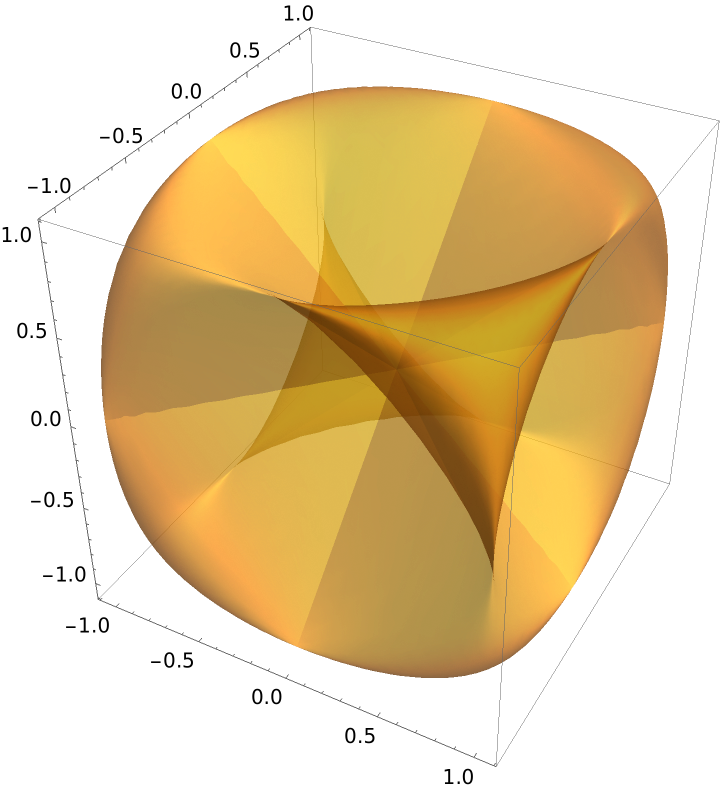The parametrization of the unit normals of the sine surface:

 In:=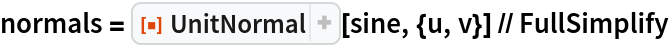Out=A Gauss map shows where the normals "live" inside the unit sphere:

 In:=Out=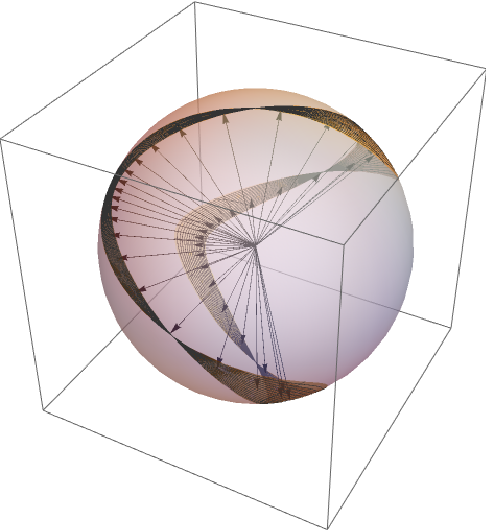Enrique Zeleny

## Version History

• 1.0.0 – 30 March 2020